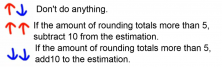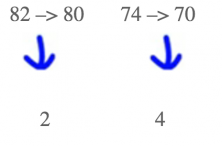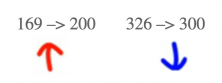Smartick is a fun way to learn math!Apr12

# Learn How to Estimate Sums with Some Examples

In this post, we are going to learn how to estimate sums as the result of an addition or subtraction. Mastering this skill is important to be able to verify if the obtained result is reasonable and the right order of magnitude.

### Estimate Sums of Amounts to The Tens:

1. Round the numbers we are going to add or subtract to the nearest tens place: if the number ends in 0, 1, 2, 3, or 4, we will round down. If the number ends in 5, 6, 7, 8, or 9, we will round up.
1. Calculate the sum of the rounded numbers.
1. Look at the amount of rounding. The amount of rounding is the number that we have subtracted or added to the original number to perform the rounding.
• If we have rounded one number up and another down, we do not have to do anything else.
• If the two numbers have both been rounded down and the amount of rounding is 5 or more, we have to add 10 to the estimate.
• If the two numbers have both been rounded up and the amount of rounding of the 2 numbers is 5 or greater, we have to subtract 10 from the estimate.Example: 82 + 74

1. We round the two numbers:

82 –> 80

74 –> 70

80 + 70 = 150

1. We look at the amount of rounding:

The two numbers are rounded down, so we look at the amount of rounding for each one:We add the two amounts of rounding:

2 + 4 = 6

Since this quantity is greater than 5, we have to add 10 to the estimate:

150 + 10 = 160

The correct estimate is 160.

### Estimate Sums of Amounts to The Hundred:

1. Round the numbers that we are going to add to the nearest hundred:
2. Calculate the sum of the rounded numbers.
3. Look at the amount of rounding.
• If we have rounded a number up and another down, we do not have to do anything else.
• If the two numbers have both been rounded down and the amount of rounding is 50 or more, we have to add 100 to the estimate.
• If the two numbers have both been rounded up and the amount of rounding of the 2 numbers is 50 or greater, we have to subtract 100 from the estimate.

Example: 169 + 326

1. We round the two numbers to the nearest hundred:

169 –> 200

326 –> 300

2. We add the two quantities:

200 + 300 = 500

3. We look at the amounts of rounding:Since one number has been rounded down and other has been rounded up, there is nothing else that has to be done.

The correct estimate of the sum is 500.

If you have enjoyed this post and want to learn more, you can review our post on How to Round Decimals.